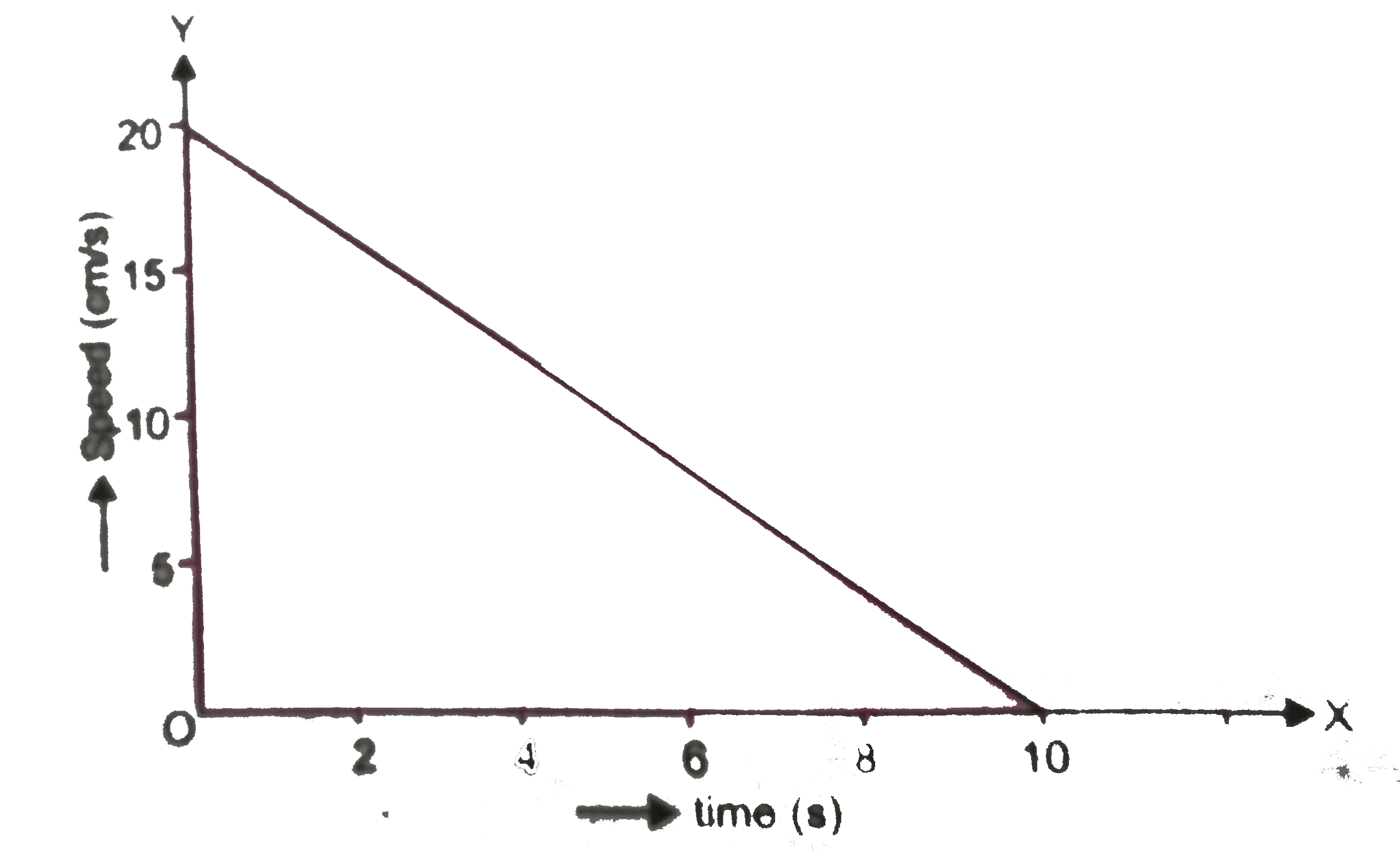# The velocity time graph of a ball of mass 20g moving along a straight line on a long table is given in (figure) How much force does the table exert

1.3k views
in Physics
closed
The velocity time graph of a ball of mass 20g moving along a straight line on a long table is given in (figure) How much force does the table exert on the ball to bring it to rest?by (66.1k points)
selected by

The initial velocity of the ball is 20 cm s^(-1). Due to the frictional force exerted by the table, the velocity of the ball decreases down to zero in 10 s. Thus, u = 20 cm s^(–1), v = 0 cm s^(-1) and t = 10 s. Since the velocity-time graph is a straight line, it is clear that the ball moves with a constant acceleration. The acceleration a is
a=(v-u)/(t)
=0 cm s^(-1) -20 cm s^(-1)//10 s
=-2 cm s^(-2)=-0.02 ms^(-2)
The force exerted on the ball F is , F=ma=(20//1000) kg xx (-0.02ms^(-2))
= -0.0004 N. The negative sign implies that the fricitonal force exerted by the table is opposite to the direction of motion of the ball.